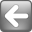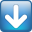## digitalmars.D.bugs - [toString] infinite loop

• Manfred Nowak (15/15) Apr 22 2005 ``` ```
• ``` Nick (45/60) Apr 22 2005 I took the time to track this one down for you. The hang occers in Unknown W. Brackets (3/21) Apr 22 2005 I've also experienced freezes with parse() and invalid formats, which ```
``` ```
```Manfred Nowak <svv1999 hotmail.com> writes: <code> import std.date, std.stdio; void main(){ static int times=[ -61367296, -36334592, -11301888] ; d_time t= parse("1 1 1970"); for( int i= 0; i < 3; i++){ toString( t + times[ i]); } } </code> Apr 22 2005Nick <Nick_member pathlink.com> writes: In article <d4aqf3\$2c5p\$1 digitaldaemon.com>, Manfred Nowak says... <code> import std.date, std.stdio; void main(){ static int times=[ -61367296, -36334592, -11301888] ; d_time t= parse("1 1 1970"); for( int i= 0; i < 3; i++){ toString( t + times[ i]); } } </code> I took the time to track this one down for you. The hang occers in std.date.toString() when called with a very large argument. It can be traced to the function YearFromTime in date.d: # int YearFromTime(d_time t) # { int y; # # // Hazard a guess # y = 1970 + cast(int) (t / (365.2425 * msPerDay)); # # if (TimeFromYear(y) <= t) # { # while (TimeFromYear(y + 1) <= t) # y++; # } # else # { # do # { # y--; # } # while (TimeFromYear(y) > t); # } # return y; # } There are two problems here. First, TimeFromYear takes an int as an argument, and passes it to DayFromYear, which both takes and returns ints. With sufficiently large parameters this calculation will overflow. But this can be considered reasonable, since years are not expected to be this big. Second, the time parameter you pass to toString is large enough that TimeFromYear(y+1) overflows but TimeFromYear(y) does not (even when all calculations are changed to use longs.) This causes the first while loop to run indefinitely. In all the test I ran (that didn't overflow, ie. with reasonable parameters), the loop count for the while/do-while loops were at most one, and mostly zero. I therefore think it's reasonably safe to add some overflow checks, ie. something like: # int count; [...] # while (TimeFromYear(y + 1) <= t) # { # if(count++ > 10) throw new Exception("Date overflow"); # y++; # } Nick Apr 22 2005"Unknown W. Brackets" <unknown simplemachines.org> writes: I've also experienced freezes with parse() and invalid formats, which may also be related. -[Unknown] <code> import std.date, std.stdio; void main(){ static int times=[ -61367296, -36334592, -11301888] ; d_time t= parse("1 1 1970"); for( int i= 0; i < 3; i++){ toString( t + times[ i]); } } </code> Apr 22 2005 <!-- google_ad_client = "pub-5628673096434613"; google_ad_width = 300; google_ad_height = 250; google_ad_format = "300x250_as"; google_ad_type = "text_image"; google_ad_channel ="7651800615"; google_color_border = "336699"; google_color_bg = "FFFFFF"; google_color_link = "0000FF"; google_color_url = "008000"; google_color_text = "000000"; //--> ```
``` ```